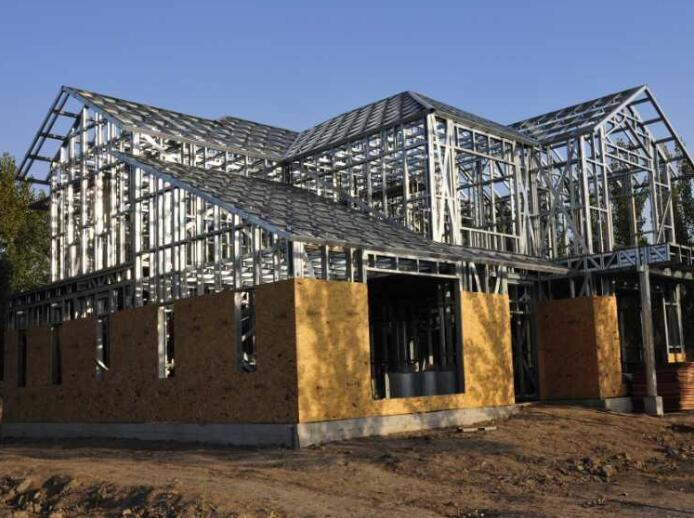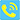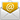﻿ steel structure house manufacturer​ and steel structure house

# Steel structure house material quality calculation

2019/10/11 10:54:01

Steel structure house material quality calculation

Quality calculation standards for different materials

Angle steel: weight per meter = 0.00785* (side width + side width - side thickness) * side thickness

Round steel: weight per meter = 0.00617 * diameter * diameter (rebar and round steel are the same)

Flat steel: weight per meter = 0.00785 * thickness * side width

Pipe: weight per meter = 0.02466 * wall thickness * (outer diameter - wall thickness)

Plate: weight per meter = 7.85 * thickness

Brass tube: weight per meter = 0.02670 * wall thickness * (outer diameter - wall thickness)

Copper tube: weight per meter = 0.02796 * wall thickness * (outer diameter - wall thickness)

Aluminum tread plate: weight per square meter = 2.96 * thickness

Non-ferrous metal specific gravity: copper plate 8.9 brass plate 8.5 zinc plate 7.2 lead plate 11.37

The calculation formula for non-ferrous metal sheets is: weight per square meter = specific gravity * thickness

The theoretical weight of steel is calculated in kilograms (kg). The basic formula is: W (weight, kg) = F (breaking area mm2) × L (length, m) × ρ (density, g/cm3) × 1/1000

The density of steel is: 7.85g/cm3,

The theoretical weight calculation formula for various steels is as follows:

Round steel wire rod (kg/m) W= 0.006165 ×d×d ,d = diameter mm

Rebar (kg/m) W= 0.00617 ×d×d ,d= section diameter mm

Square steel (kg/m) W= 0.00785 × a ×a , a= edge width mm

Flat steel (kg/m) W= 0.00785 ×b ×d , b= side width mm, d= thickness mm

Hexagonal steel (kg/m) W= 0.006798 × s × s , s = distance to the side mm

Octagonal steel (kg/m) W= 0.0065 × s × s , s = distance to the side mm

Equilateral angle steel (kg/m) = 0.00785 × [d (2b – d ) + 0.215 (R2 – 2r 2 )] : b= edge width, d = edge thickness, R = inner arc radius, r = end arc radius

Unequal angle steel (kg/m) W= 0.00785 ×[d (B+b – d )+0.215 (R2 – 2 r 2 )] : B= long side width, b= short side width, d= side thickness, R = inner arc radius r = end arc radius.

Channel steel (kg/m) W=0.00785 ×[hd+2t (b – d )+0.349 (R2 – r 2 )] h= high, b= leg length, d= waist thickness, t= average leg thickness, R = inner arc radius, r = end arc radius

I-beam (kg/m) W= 0.00785 ×[hd+2t (b – d )+0.615 (R2 – r 2 )] h= high, b= leg length, d= waist thickness, t=average leg thickness, R= inner arc radius, r=end arc radius

Steel pipe (including seamless steel pipe and welded steel pipe (kg/m) W= 0.02466 × S (D – S), D = outer diameter, S = wall thickness.we are steel structure house manufacturer and provide steel structure house,we can guarantee quality.

Mainly have the following characteristics:

1)Adopt high-efficiency lightweight thin-walled profiles, light weight, high strength and small footprint.

2)The structure of the accessories is automatic, continuous and high-precision production. The product specifications are serialized, finalized and matched. Each part is dimensionally accurate.

3)Structural design, detailed design, computer simulation installation, factory manufacturing, site installation, etc. are synchronized in a short time difference.

4)Basic dry work does not have wet work, and interior decoration is easy to be in place at one time. Lightweight steel is galvanized and coated with a beautiful appearance and anti-corrosion, which helps to reduce the cost of enclosure and decoration.

5)It is convenient to enlarge the column spacing and provide more separation space, which can reduce the height of the floor and increase the building area (the utility space can reach 92%). The advantages in layering, transformation and reinforcement are obvious.we are steel structure house manufacturer and provide steel structure house,we can guarantee quality.+8615081877521nancy@wzhgroup.com